Latest Banking jobs   »

# Quantitative Aptitude Quiz For IDBI AM/Executive 2022- 14th June

Q1. Ritu and Anu together can do a work in 16 days where as Anu alone can do it in 24 days. If Neha alone can do the same work in 30 days, then find the ratio of efficiency of Neha to efficiency of Ritu?
(a) 5 : 8
(b) 8 : 5
(c) 5 : 3
(d) 2 : 3
(e) 7 : 8

Q2. A mixture contain Iron and copper in the ratio of 7: 12. When 30 kg Iron and 40 kg copper mixed into the mixture the ratio becomes 5 : 8. Find the ratio of the initial quantity of Iron to the final quantity of copper.
(a) 9 : 8
(b) 5 : 3
(c) 3 : 16
(d) 7 : 16
(e) 8 : 7

Q3. A shopkeeper have 800 kg of Sugar, a part of which he sells at 10% profit and remaining at 15% loss thus, he incurred overall loss of 5.625%. What would be the profit/loss percentage, if he interchanges the quantity he sold initially.
(a) 1.5%
(b) 0.375%
(c) 1.125%
(d) 2.125%
(e) 0.625%

Q4. In how many ways the word “Examination” can be arranged so that all consonant comes together?
(a) 14400
(b) 28800
(c) 720
(d) 75600
(e) 50400

Q5. Muskaan gives 20% of her salary to Simran. Simran gives 40% of this money to her Mom and remaining money is invested for 2 years in a scheme which offer Compound Interest at the rate of 20% p.a. If Interest earned on amount invested is Rs6600 then, find the salary of Muskaan.
(a) 150000
(b) 175000
(c) 140000
(d) 125000
(e) 120000

Q6. Difference between the compound interest and simple interest earned on a certain amount in 2 years at the rate of 12 % p.a. is Rs 144. If same amount is invested in scheme ‘P’ which offer simple interest at the rate of 15% p.a. for 4 years then, find the simple interest earned from scheme ‘P’.
(a) 4000
(b) 6000
(c) 14400
(d) 4500
(e) 4800

Q7. From a well-shuffled pack of a cards, a card is picked out, if it is a face card then a coin is tossed. Find the probability of getting a head.
(a) 3/26
(b) 5/13
(c) 7/26
(d) 8/13
(e) 7/13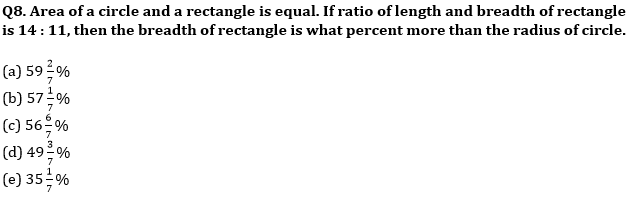Q9. Ram is 2 years older than Shyam while Shyam is 4 years older than Akshay. If ratio of Ram’s age 6 year hence to Shyam’s age 2 year ago is 17 : 12 then, find Akshay’s age 8 years hence ?
(a) 34
(b) 36
(c) 32
(d) 28
(e) 30

Q10. Raman is travelling with a speed of 15 km/hr and reached point ‘X’ at 12:00 pm. If he had travelled with a different speed he would reach ‘X’ at 3 : 00 pm but if he further increased its changed speed by 80% then, he reach ‘X’ at 11 : 00 am. Find the speed with which Raman reach ‘X’ at 11 : 00 am.
(a) 10
(b) 20
(c) 15
(d) 18
(e) 16

Q11. Efficiency of B is two times more than efficiency of A. Both started working alternatively, starting with B and completed the work in total 37 days. If C alone complete the same work in 50 days then find in how many days A and C together will complete the work?
(a) 24 days
(b) 30 days
(c) 36 days
(d) 48 days
(e) 18 days

Q12. Abhishek lent Satish Rs.12000 on C.I. at the rate of 20% per annum and at the end of first year Satish borrowed Rs.x more from Abhishek on C.I. at the same rate. If at the end of second year, Satish paid total amount of Rs.20400 to Abhishek then find how much extra amount Satish borrow at the end of first year?
(a) Rs.2400
(b) Rs.2000
(c) Rs.3600
(d) Rs.2600
(e) Rs.4000

Q13. In a River there are two boats A and B, where boat A covers 30 km downstream and boat B covers 30 km upstream. Boat B takes 2 hours more than boat A in covering the given distance. If sum of speed of boat A in still water and boat B in still water is 16 km/hr and speed of water current is 1 km/hr then find the speed boat B in still water?
(a) 8 km/hr
(b) 4 km/hr
(c) 5 km/hr
(d) 6 km/hr
(e) 7 km/hr

Q14. Veer and Subham entered into partnership. Veer invested Rs.3x for first four month and Rs.5x for next six months and Subham invested Rs.1800 for 12 months. If Veer and Subham got profit share in the ratio of 7 : 9 then, find the value of ‘5x’ ?
(a) 2000 Rs.
(b) 1600 Rs.
(c) 2400 Rs.
(d) 3600 Rs.
(e) 4000 Rs.

Q15. A vessel contains mixture of tin and copper in the ratio of 2 : 3. Some amount of mixture is taken out and 28 gm copper is added to the remaining mixture so that amount of copper becomes 66 ⅔% in the new mixture. If 12 ½ % of initial mixture is 22.5 gm then, find what amount of tin was taken out from the initial mixture?
(a) 16gm
(b) 14gm
(c) 12gm
(d) 10gm
(e) 18gm

Solutions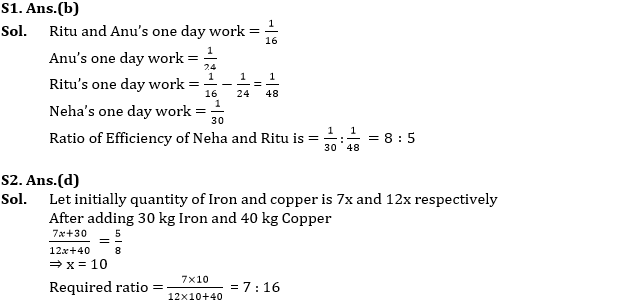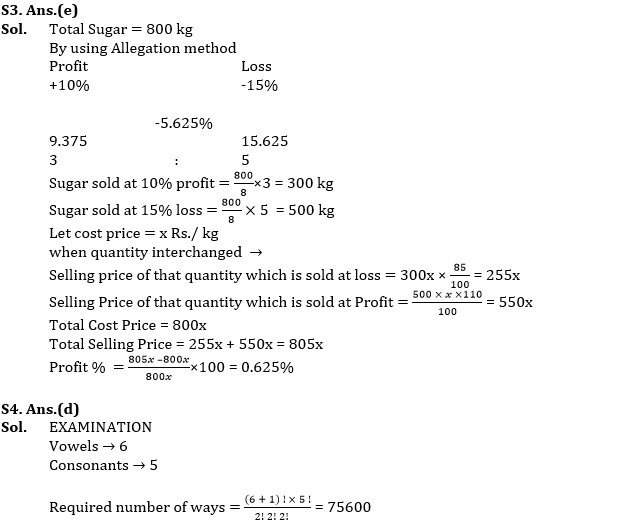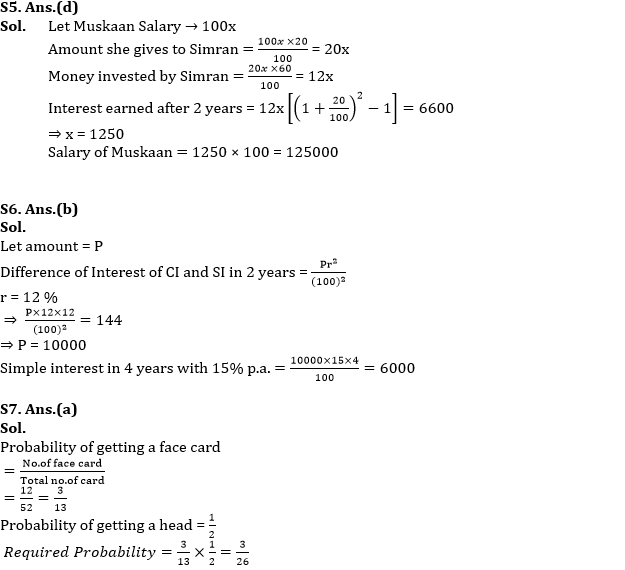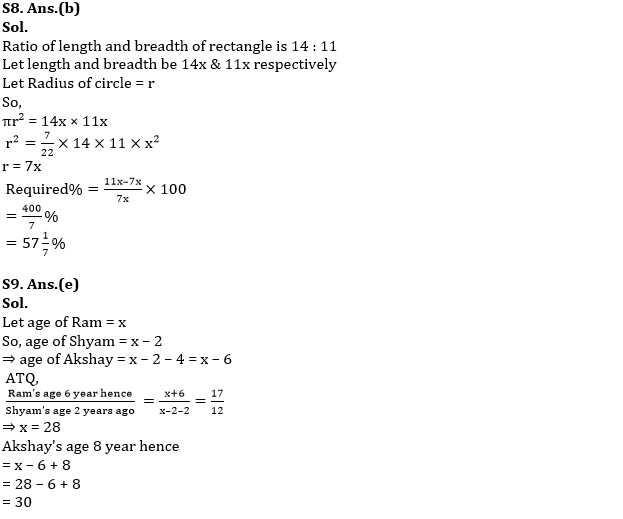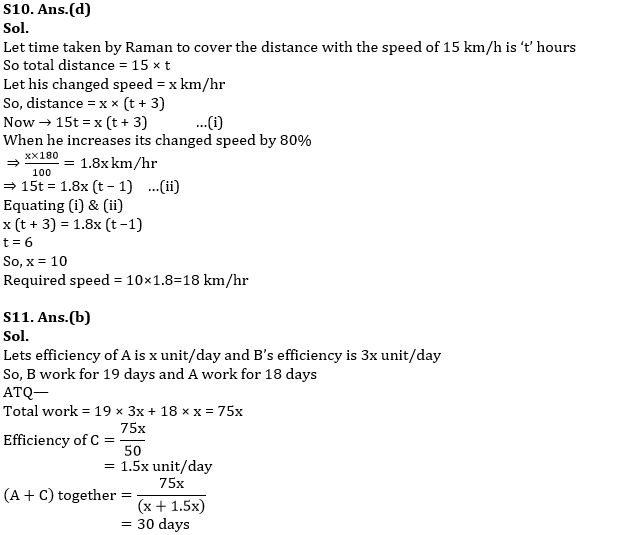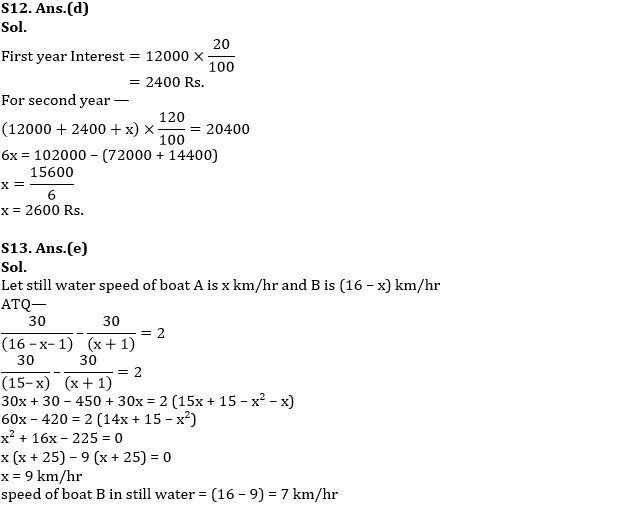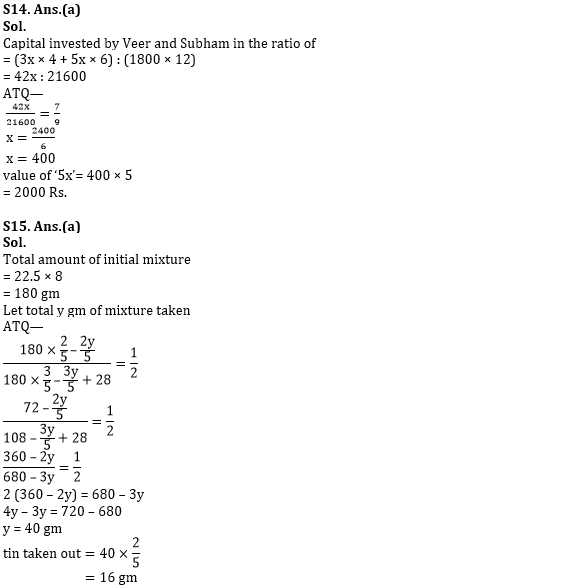#### Congratulations!